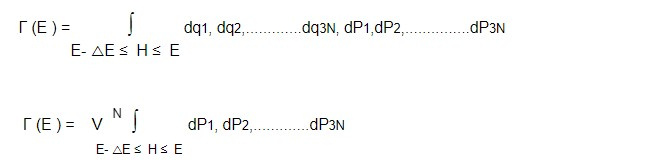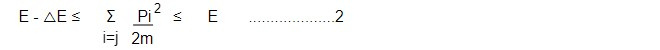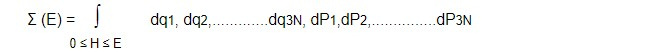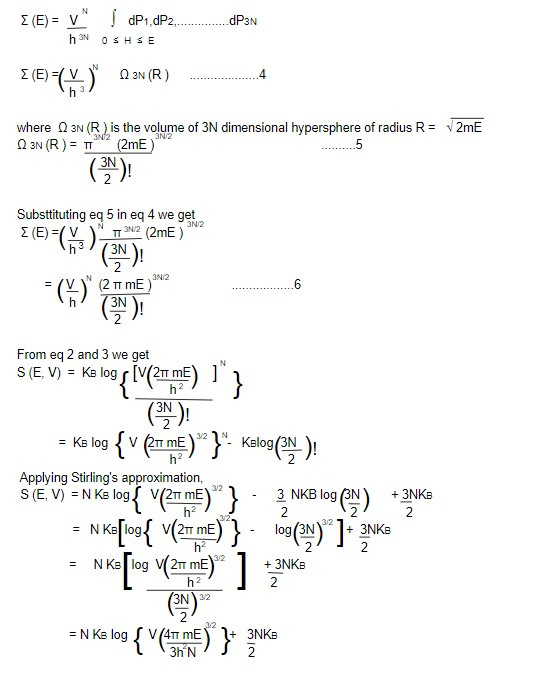# Entropy of a classical ideal gas using the concept of microcanonical ensemble

The microcanonical ensemble describes a system that is isolated and has a fixed total energy. For an ideal gas, the total energy of the system is the sum of the kinetic energies of all its constituent particles.

Consider a microcanonical ensemble for a system of 'N' non interacting point particles of mass M confined in a volume 'V' with total energy △E and E.

The volume of microcanonical ensemble in phase space is given bywhere momentum space integral is to be evaluated subjected to constraint,The range △E can be replaced by the range 0 to E. So,

S(E, V) = KBlog Γ (E) can be expressed as

S(E, V) = KBlog Σ (E) ..................3Σ (E) is dimensionless so we introduce a constant having dimension of momentum X distanceThis is the required expression for entropy of a classical ideal gas.

This note is taken from Statistical Mechanics, MSC physics, Nepal.

This note is a part of the Physics Repository.

Next topic: Gibb's paradox and its resolution